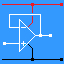RANDOM PAGE

SITE SEARCH

LOG
IN

HELP

# Reactance

This is the AQA version closing after June 2019. Visit the the version for Eduqas instead.

Reactance is measured in Ohms and is equivalent to resistance but it applies to Alternating Current circuits.

Reactance depends on the frequency (F) of the AC. Resistance remains constant at all frequencies.

Reactance applies to Inductors and Capacitors.

INDUCTORS:    XL = 2 π F L    where L is the inductance measured in Henrys.

CAPACITORS:    XC = 1 / (2 π F C)    where C is the capacitance measured in Farads.

In RC filter circuits, the cut-off or breakpoint frequency occurs when the resistance of the resistor is equal to the reactance of the capacitor.

In LC tuned circuits, the resonant frequency occurs when the reactance of the inductor and the capacitor are equal.

The more advanced maths for reactance is beyond A Level standard and it involves vector diagrams or calculations using imaginary numbers.

reviseOmatic V3     Contacts, ©, Cookies, Data Protection and Disclaimers Hosted at linode.com, London